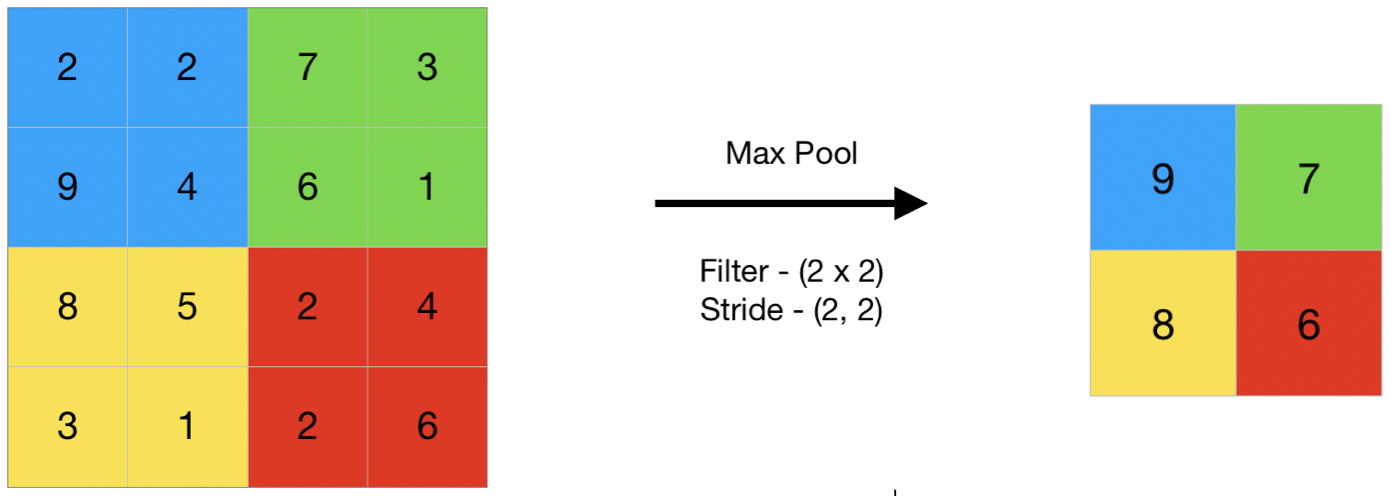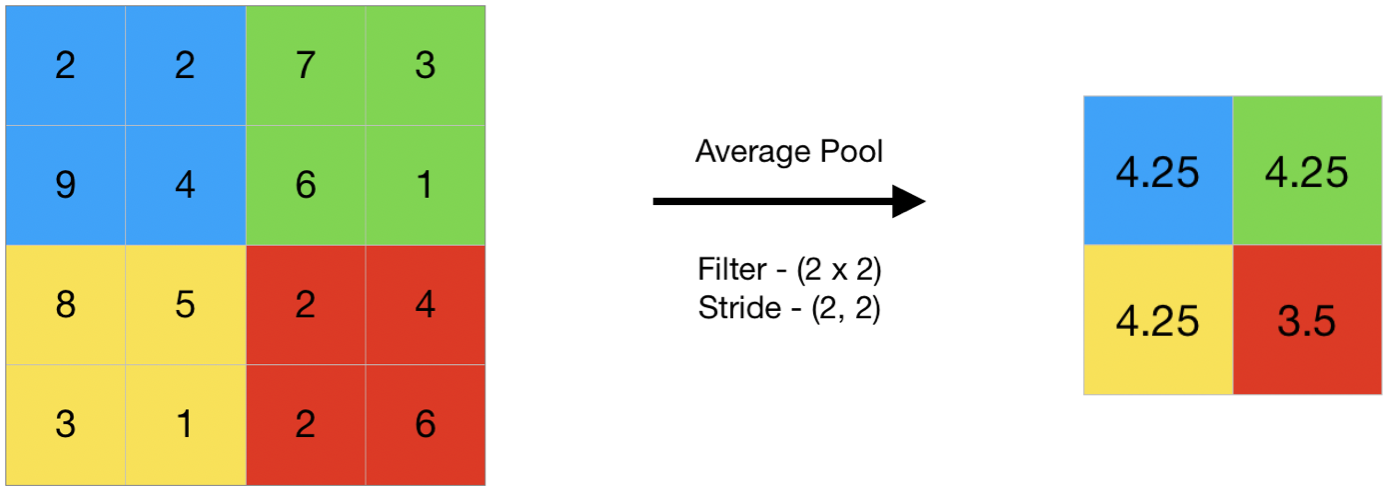# CNN | Introduction to Pooling Layer

• Difficulty Level : Basic
• Last Updated : 24 Aug, 2022

The pooling operation involves sliding a two-dimensional filter over each channel of feature map and summarising the features lying within the region covered by the filter.
For a feature map having dimensions nh x nw x nc, the dimensions of output obtained after a pooling layer is

`(nh - f + 1) / s x (nw - f + 1)/s x nc`

where,

```-> nh - height of feature map
-> nw - width of feature map
-> nc - number of channels in the feature map
-> f  - size of filter
-> s  - stride length```

A common CNN model architecture is to have a number of convolution and pooling layers stacked one after the other.

#### Why to use Pooling Layers?

• Pooling layers are used to reduce the dimensions of the feature maps. Thus, it reduces the number of parameters to learn and the amount of computation performed in the network.
• The pooling layer summarises the features present in a region of the feature map generated by a convolution layer. So, further operations are performed on summarised features instead of precisely positioned features generated by the convolution layer. This makes the model more robust to variations in the position of the features in the input image.

#### Types of Pooling Layers:Max Pooling

1. Max pooling is a pooling operation that selects the maximum element from the region of the feature map covered by the filter. Thus, the output after max-pooling layer would be a feature map containing the most prominent features of the previous feature map.1. This can be achieved using MaxPooling2D layer in keras as follows:
Code #1 : Performing Max Pooling using keras

## Python3

 `import` `numpy as np``from` `keras.models ``import` `Sequential``from` `keras.layers ``import` `MaxPooling2D`` ` `# define input image``image ``=` `np.array([[``2``, ``2``, ``7``, ``3``],``                  ``[``9``, ``4``, ``6``, ``1``],``                  ``[``8``, ``5``, ``2``, ``4``],``                  ``[``3``, ``1``, ``2``, ``6``]])``image ``=` `image.reshape(``1``, ``4``, ``4``, ``1``)`` ` `# define model containing just a single max pooling layer``model ``=` `Sequential(``    ``[MaxPooling2D(pool_size ``=` `2``, strides ``=` `2``)])`` ` `# generate pooled output``output ``=` `model.predict(image)`` ` `# print output image``output ``=` `np.squeeze(output)``print``(output)`1. Output:
```[[9. 7.]
[8. 6.]]```

### Average Pooling

1. Average pooling computes the average of the elements present in the region of feature map covered by the filter. Thus, while max pooling gives the most prominent feature in a particular patch of the feature map, average pooling gives the average of features present in a patch.1. Code #2 : Performing Average Pooling using keras

## Python3

 `import` `numpy as np``from` `keras.models ``import` `Sequential``from` `keras.layers ``import` `AveragePooling2D`` ` `# define input image``image ``=` `np.array([[``2``, ``2``, ``7``, ``3``],``                  ``[``9``, ``4``, ``6``, ``1``],``                  ``[``8``, ``5``, ``2``, ``4``],``                  ``[``3``, ``1``, ``2``, ``6``]])``image ``=` `image.reshape(``1``, ``4``, ``4``, ``1``)`` ` `# define model containing just a single average pooling layer``model ``=` `Sequential(``    ``[AveragePooling2D(pool_size ``=` `2``, strides ``=` `2``)])`` ` `# generate pooled output``output ``=` `model.predict(image)`` ` `# print output image``output ``=` `np.squeeze(output)``print``(output)`

1. Output:
```
[[4.25 4.25]
[4.25 3.5 ]]```

### Global Pooling

1. Global pooling reduces each channel in the feature map to a single value. Thus, an nh x nw x nc feature map is reduced to 1 x 1 x nc feature map. This is equivalent to using a filter of dimensions nh x nw i.e. the dimensions of the feature map.
Further, it can be either global max pooling or global average pooling.
Code #3 : Performing Global Pooling using keras

## Python3

 `import` `numpy as np``from` `keras.models ``import` `Sequential``from` `keras.layers ``import` `GlobalMaxPooling2D``from` `keras.layers ``import` `GlobalAveragePooling2D`` ` `# define input image``image ``=` `np.array([[``2``, ``2``, ``7``, ``3``],``                  ``[``9``, ``4``, ``6``, ``1``],``                  ``[``8``, ``5``, ``2``, ``4``],``                  ``[``3``, ``1``, ``2``, ``6``]])``image ``=` `image.reshape(``1``, ``4``, ``4``, ``1``)`` ` `# define gm_model containing just a single global-max pooling layer``gm_model ``=` `Sequential(``    ``[GlobalMaxPooling2D()])`` ` `# define ga_model containing just a single global-average pooling layer``ga_model ``=` `Sequential(``    ``[GlobalAveragePooling2D()])`` ` `# generate pooled output``gm_output ``=` `gm_model.predict(image)``ga_output ``=` `ga_model.predict(image)`` ` `# print output image``gm_output ``=` `np.squeeze(gm_output)``ga_output ``=` `np.squeeze(ga_output)``print``(``"gm_output: "``, gm_output)``print``(``"ga_output: "``, ga_output)`

1. Output:
```
gm_output:  9.0
ga_output:  4.0625 ```

My Personal Notes arrow_drop_up